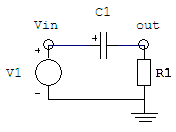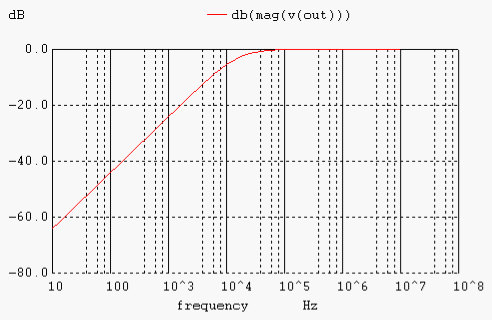(WinSpice and Scilab interface)

In this article we present a Scilab routine to drive the simplest high-pass filter designed in the WinSpice simulator. This method can also be easily adapted to simulate and drive any circuit entered in SPICE. SPICE stands for Simulation Program with Integrated Circuit Emphasis.

The concept of the Scilab driver is illustrated by simulating a very simple RC high-pass filter. In this example we're using specifically Scicoslab ver. 4.3 (Scilab compatible) and WinSpice3 ver. 1.04.07.

Interchanging information between Scilab and WinSpice allows the implementation of optimization techniques and graphical possibilities not included in the simulator environment itself. The shown approach is very flexible and utilizes the inherent capability of this SPICE simulator to include  text files with parameters and print data to external .csv (comma separated values) files.

This is the general idea...

• First, the circuit in Spice is designed and defined (.cir file). This circuit is simulated and tested within the WinSpice environment before any attempt is made to interact with Scilab. We separate the parameters or components of interest and include them in another text file (using the .include directive in the main file).
• Second, we write the output of the simulator to an external file, that is, we include 'write' commands in the main .cir file so that the results are saved as external (.csv) text files that can be read by Scilab.
• Third, now we just have to write parameters, run the simulation and read the response. All this can be done from/by Scilab as if the simulator was an ordinary built-in function to be called!

Now, let's see the details with a specific example...

First Step

We create, test and simulate this simple RC high-pass filter in WinSpice:We initially (arbitrarily) use these values:
R = 10k ohms, C = 1 nF

The main .cir file (which is also a text file) is:

---- RC - High Pass Filter ----

V1  Vin  0   dc 0   ac 1

.include param_hpf.txt
R out 0 {res1}
C vin out {cap1}

.control
AC DEC 10 10 1MEGHZ
plot vdb(out)
.endc
.end

The actual parameters are located in a separate file named ‘param_hpf.txt’, which in this case contains one line only:

.param res1= 1.000000e+004 cap1= 1.000000e-009

As expected, the frequency analysis delivers this result:Second Step

We include appropriate 'write' commands in the main .cir file, so that the results are saved as .csv files available to Scilab. The 'quit' command allows us to close the simulator window.

The control block changes into:

.control
AC DEC 10 10 10MEGHZ
plot vdb(out)
write RC_HPF_ac_out.csv vdb(out)
quit

.endc

Third Step

We now create a function to be run by Scilab to write the text file that includes the parameters, launch the simulator and read the results. This is the code to accomplish it:

function [x, y] = WS_high_pass_filter(p)
// Create and save the file 'param_hpf.txt' to be included in
// the circuit to be run by WinSpice.
[fd, err] = mopen('param_hpf.txt', 'w');
n = mfprintf(fd, "%s %e %s %e \n", '.param res1=', p(1), 'cap1=', p(2))
mclose(fd);

// Run WinSpice
// Make sure wspice3.exe and all the involved files are available
// in this directory
host('wspice3 high_pass_filter.cir')

// Read data saved in the .csv file
[x, y] = getWSpicedata('RC_HPF_ac_out.csv');
endfunction

There is an important portion of additional code to read the .csv files.

function [x, y] = getWSpicedata(f);
// f = name of the .csv file
// x = frequencies
// y = response of the circuit in dB

// We open the file and discard the first row
[fd, err] = mopen(f, 'r');
s = mgetl(fd, 1);

// We start reading all the rows, we read them as strings
// We keep on reading until we reach an empty row
ix = 1;
while ~meof(fd)
s = mgetl(fd, 1);
if s == []
break
end
// 'strindex' finds the position of all the commas in the string
k = strindex(s, ',');
// 'part' extract a portion of the string
// 'eval' converts the string into a number
// we take only the first and the third columns
x(ix) = eval( part(s, 1 : k(1)-1) );
y(ix) = eval( part(s, k(2)+1 : k(3)-1) );
ix = ix + 1;
end
mclose(fd);
endfunction

Now, we're ready to perform some simulations driving WinSpice from Scilab.

xdel(winsid()); clear; clc

// We declare our functions to drive the circuit
getf('WS_high_pass_filter.sci')
getf('getWSpicedata.sci')

// We decide our parameters
p = [10e3 1e-9];

// This function calls WinSpice and reads the results
[x y] = WS_high_pass_filter(p);

// We now plot the response of the circuit
plot2d(x, y, logflag="ln")
xgrid

For R1 = 10k ohms and C1 = 1 nF, we get this AC response in dB.For R1 = 1k ohms and C1 = 1 uF, we run it like this...

p = [1e3 1e-6];
[x y] = WS_high_pass_filter(p);

This is the new AC response in dB:We can now use WinSpice as if it was another Scilab or Scicoslab function! This means that we can work through circuit optimization using functions such as leastsq, datafit, optim, and others.

The main idea is that now we can try some numbers in our design, we can simulate the results and use Scilab to modify relevant values in the circuits, iteratively in optimization routines.

Maybe you'd like to see a similar example on a low-pass filter in Matlab.

Reference: